# How to Draw a Five-Dimensional Cube

### Copyright (c) 2004 by Gary Felder

This paper introduces a method I developed for representing objects in more than three dimensions. With a little practice you can learn to visualize objects like 5D spheres, 4D cubes, and so on, and even see what happens when you rotate them in different ways.

This method is original in the sense that I thought of it. I am quite confident that it's not original in the sense that many other people have doubtless thought of it and written about it before. I have, quite frankly, never bothered to look. The purpose of this paper is not to lay first claim to these ideas, but simply to share them with anyone else who might find them fun to play with.

### The Basic Idea

In his delightful novel Flatland, Edwin Abbott describes a race of beings who live in two dimensions. All of the characters in the book have simple geometrical shapes, which makes the presentation of the ideas clearer. The main character, a square, is visited by a sphere from the third dimension. Being confined to his flat plane, however, the square can only see the part of the sphere that is crossing his 2D world: "the Sphere [...] manifested himself to me, or to any Flatlander, as a Circle." This example illustrates the basic dilemma I'm addressing. As 3D beings we can only see, or even visualize, objects in three or fewer dimensions. In Abbott's book the main character is eventually knocked off his planar world and is temporarily able to see 3D objects directly. He later speaks of the wonders he saw, which he "found daily more difficult to reproduce even before my own mental vision." I maintain that even if we were somehow put in a 4D world our eyes could no more see a 4D object than Abbott's character could have seen off of his plane in the first place. Whatever we might or might not see in such a world, however, I will take it as a given that living as we do in a 3D world we can not physically picture anything higher-dimensional than ourselves.

So what's the trick? The trick is that we can use the images we can picture (1D, 2D, or 3D) to represent higher-dimensional objects. Before saying what I mean by that, I want to quickly say what I don't mean. A good artist can easily use a 2D image to represent a 3D object, with proper perspective, shading, and so on, often to the point of fooling the viewer. I am not talking about that technique since it relies on our innate ability to visualize 3D objects. I will make use of that at certain points, showing some conventional 3D images of spheres and the like, but that's not the point of this paper.

The method I am describing makes use of multiple slices of the objects we wish to represent. To see how this works I can start with a simple example: using 2D images to represent a 3D sphere. As Abbott described, each horizontal slice through a sphere looks like a circle, with the radii getting successively bigger and then smaller again as you move up. We can draw the sphere by showing a representative sample of such images, like so: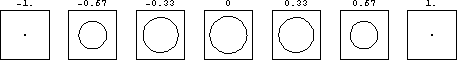The label above each image shows the z-coordinate, or height, of the image. To reconstruct the actual sphere in 3D we have to imagine all of these images stacked on top of each other, something a Flatlander could never do.

Of course this series of 2D snapshots doesn't contain all the information about the sphere, but by using increasing numbers of slices you can represent the object to arbitrary accuracy. In this case seven images are perfectly adequate to capture all the important information about the sphere. In particular we can easily interpolate between these images to imagine what any intermediate slice would look like.

So far I've only shown you how to represent a 3D sphere, which we could easily have done anyway. This method, however, allows us to represent an object in 4D or more as well. Consider a 4D hypersphere, i.e. an object consisting of all the points in a 4D space equidistant from a single point:Just as a 3D sphere could be represented by showing the 2D slices through it at different heights z, so the 4D hypersphere can be represented by showing the 3D slices through it at different values of the fourth dimension.

To make this idea clearer I'm going to spend an entire section talking about using 2D images to represent 3D objects. Hopefully by starting out with objects that you can picture such as spheres, cubes, and lines in 3D, you will be able to get a clear idea of how this system works. Then I'll move on to talking about representations of 4D objects using 3D slices. In the next two sections after that I will talk about some ways you can use this technique to answer questions about higher-dimensional objects like how many edges a hypercube has or how to rotate it in different directions. Then I'll briefly talk about how to extend these ideas to 5D objects and beyond.

### 3D Objects Represented With 2D Slices

Start by considering the simplest of shapes: a line. To make life even simpler let's start with a vertical line going through the origin. (In a conventional plot this line would be the z-axis.) What do horizontal slices through this line look like? They all look like points, sitting at the origin of the xy plane.(The ellipsis—set of three dots—at each end is simply to illustrate that the slices go on forever since the line is infinite.) This drawing is the simplest example of the technique I'm describing so make sure you understand it before you move on. All of these slices are identical. To a flatlander every slice shows a single point at the exact same place, namely x=y=0. We know that what makes them different is that each one is actually at a different height z, but the flatlander simply has to imagine each one sitting at the same point in his plane and take it on faith that they are separated from each other in a third dimension that he can't visualize.

Of course there are other lines that we can draw. We can consider a horizontal line such as the x-axis:(In this case I didn't put dots on the ends. Even though I could draw more slices, they would all be blank. In general I will use the triple dots to indicate objects like the z-axis where the object shows up in every slice. I won't use them for objects confined to a finite number of slices, like the x-axis here or the sphere in the previous section.) In 3D the vertical and horizontal lines look very similar, but to the flatlander they couldn't look more different. The flatlander can recognize the slice at z=0 as containing an ordinary line, and all of the other slices are empty.

What about a tilted line? Imagine a diagonal line tilted at 45 degrees between the x and z axes. (This is the line x=z, y=0.) Once again each horizontal slice looks like a single point, only now each point has a different value of x.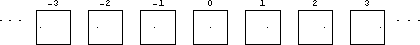Remember that these drawings show the (x,y) plane and the labels show the value of z. Try to picture this diagonal line in 3D as you look at this image. (I would have put an image of the line in 3D next to the slices but I've found that a 3D plot with just a line looks confusing; you can't tell which way the line is supposed to be pointing. So I'm going to rely on your ability to picture this line in 3D without an image.) You should be able to convince yourself that every horizontal slice through the line hits it at exactly one point. However, slices at different heights (values of z) hit the line at different places (values of x), so the slices look as shown above.

If you're with me so far, congratulate yourself. You have the basic idea, and everything else consists of applying it to more complicated situations. To start with, let's consider a few basic 3D shapes and see what they look like in 2D slices. For the rest of this section I will draw each image in two ways: as a regular 3D drawing, and as a series of 2D slices. This is to help you see how the two relate to each other so you can get used to the idea. When I go on to talk about 4D images we won't have that luxury because I can only draw the 4D images as slices.

Let's start with a cube. Imagine we have a cube centered on the origin with its faces parallel to the x, y, and z axes, as shown below. The horizontal slices through this cube are squares, each centered on the origin of the xy plane.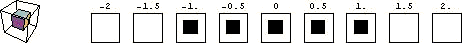I could have included more slices—values of z—and each one between -1 and 1 would have shown a square, while each one outside this range would have been empty. Before moving on make sure that you can clearly see how the 3D image on the left corresponds to the 2D slices on the right. (Note that in this as in all 3D images in this paper the axes are tilted so that you can see the shape more clearly.)

To conclude this section we can return to our original example, the sphere that appeared to our hapless Flatlander. As noted above, each horizontal slice through the sphere appears as a circle. However, the size of that circle depends on the height (z value) of the slice.### 4D Objects Represented With 3D Slices

Now we can extend the method to represent 4D images, which we couldn't otherwise draw, by using 3D slices. In four dimensions we need another coordinate in addition to our usual (x,y,z). I will call this coordinate a.

Let's start by considering a plane. The easiest way to draw a plane in a 4D space is to choose one that lives entirely in one 3D slice, such as the plane a=z=0Just as the labels above our 2D slices in the last section represented the value of z in each slice, the labels above these 3D slices represent the value of a in the slice.

Recall that when we were drawing lines in 3D using 2D slices, we saw that the slices for the x-axis (y=z=0) looked very different from the slices for the z-axis (x=y=0). That's because the x-axis only showed up in one slice, whereas the z-axis extended to different values of z. In a similar vein, we can now consider the plane x=z=0, which exists at all different values of a. At each value of a the slice through this plane consists of a lineThink back to our discussion of the z-axis in the previous section. We said there that each slice looked identical, simply a point at the origin of the 2D slice. A flatlander would simply have to imagine that those points weren't really all in the same spot, but were at different "heights" in a dimension (z) that the flatlander couldn't picture. We are now in that same position. All of the 3D slices for this plane look the same, but to put them together into a full 4D object we have to imagine that they are all "on top of each other" in a fourth dimension.

What about a tilted plane such as x=z=a? For each value of a this is once again a line, but now each slice is a different lineEach of these slices shows a line going into and out of the page (parallel to the y-axis), but in each successive slice the line is higher up and further to the right. As different as they look in this representation, this and the two previous images are all ways of showing ordinary 2D planes in a 4D space.

Just as a 3D space can contain points, lines, and planes, a 4D space should be able to contain points, lines, planes, and 3D spaces. What does a 3D space look like? Once again the simplest case is when the 3D space is contained entirely in one slice. In this case each slice is a complete 3D space, defined by the condition a=some constant. For example a=0 is the 3D space consisting of the slice in the center of our plots. (I won't draw this one, but you get the idea.)

However, z=0 is also a 3D space. In each slice this space looks like a plane at z=0.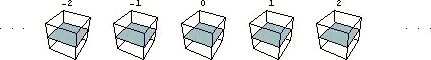Just as we were able to tilt a line or a plane, we can also tilt a 3D space. We can define another space, for example, by the condition z=a. In this case each slice is once again a horizontal plane, but each such plane is at a different height.In general you can construct any 3D space by starting with a plane (any plane you like) in one of your slices and then moving that plane by the same fixed amount from each slice to the next successive one.

All of this is cute, but you may feel that having shown a hypercube as a grid of images of cubes doesn't actually tell you anything more about it than you would have known without the picture. After all, one of the great uses of drawing pictures (or holding mental images) of shapes and objects is that we can easily figure things out about them that would be very difficult to do algebraically. In this section I give several examples to try to convince you that constructing 4D and 5D images in this way does allow you to answer questions that would be hard to answer otherwise. I will not try to convince you that such questions are interesting to think about in the first place; I'll just blithely assume that if you've made it this far you probably agree with me on that one.

As a first example, let's consider a 4D hypercube. If someone asks you how many sides a square or a cube has, you can easily picture one and count to get the answers four and six respectively. Does the progression continue like that? How many sides does a 4D hypercube have?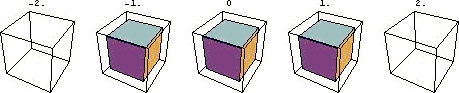To answer this we have to be clear what we mean by a side. Thinking about the square and cube, we can see that the "side" of an N-dimensional object is an (N-1)-dimensional shape that bounds the object. In the case of a square the sides are line segments. In the case of a cube they are squares. (This same definition applies to other shapes such as pentagons or pyramids.) So the sides of our 4D hypercube should be cubes.

Looking at our picture it might seem at a glance that the hypercube is bounded only by two cubes. It's true that there are two cubes at a=-1 and a=1 that bound the hypercube, but these aren't the only ones. Sometimes a cube just looks like a cube, but sometimes a cube looks like a series of images of squares. Consider the object made up of the tops of the cubes in each of the three images shown above. This object stretches from -1 to 1 in the x, y, and a directions. In other words it's a regular 3D cube, and it forms the boundary of our hypercube because it represents the highest z values—in a sense, the "top" of the hypercube. Bearing this in mind, we can readily see that there are in fact eight cubes bounding our hypercube: two that look like normal cubes to us and six that look like stacks of images of squares.

This example illustrates the fact that, although the a dimension is mathematically just the same as the others, the way we are drawing it makes it look very different. Thus some cubes appear like cubes and others appear like stacks of squares.

As another example of a question we can address with this technique, consider the old high school geometry dictum that two points define a line and three points define a plane. I remember thinking when I learned that rule that it seemed that four points should define a 3D space, and yet it's not immediately obvious what that even means. Once you start picturing your 3D space inside a 4D or 5D space, however, this assertion is perfectly meaningful and can be easily checked. (I don't remember this for sure, but I think it might have been this question that led me to develop these ideas in the first place.)

To test this idea, let's start by considering three points in a 4D space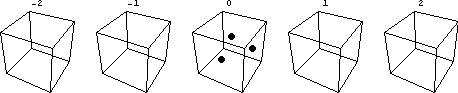Clearly these three points all lie in the 3D space at a=0. Is there any other space that intersects all three points? I encourage you to stop and try to answer that for yourself before reading on. Recall that 3D spaces can look like two very different things. The space at a=0 just looks like a regular 3D space. The space at, say, z=0, however, looks like a series of planes. The three points above all clearly lie at a=0. If they also happen to be at z=0 (not obvious one way or another from the drawing) then we can construct a second space through them by considering the union of the z=0 plane in every a=constant frame.

We've thus shown that there are two spaces that can go through these three points. Shouldn't there be an infinite number of them, though, just like there are infinitely many planes that can go through any two points? There are, as it turns out. We're supposing that these three points lie in the (a=z=0) plane. In addition to the space defined by the set of planes z=0 at every value of a, there is another space defined by z=a. This space looks like a stack of planes that move up or down in your image as you move along the a dimension.There's also a space defined by z=-a, and one by z=3a, and so on. Since all of these spaces intersect the plane a=z=0, they all contain the points shown above. We can thus conclude that three points are not sufficient to define a space.

What about four points? If we add a fourth, arbitrary point, it will in general pick out a unique space from the set described above. The plot below shows four points and the space defined by them.Note that there was nothing special about the fact that three of these points lie at a=0. I leave it as an exercise to the reader to think about what the space defined by four points looks like if those four points are scattered completely randomly throughout the 4D space. You can also convince yourself that in 5D it still takes exactly four points to define a 3D space, just like two points defines a line equally well in 2D or 3D, or any higher number of dimensions. Finally, I should remind you that "Three points defines a plane" needs to have the caveat that the three points are not collinear. Similarly, four coplanar points do not uniquely define a space. Think about it.

I'll close this section with one more exercise for the reader, to make sure you've got the idea. In 2D I can draw a square and then draw a circle around it centered at the same point that just barely touches the square.The circle touches the square at four points, namely the four corners. Similarly I could draw a cube and surround it by a sphere just big enough to barely touch the cube and I would find that they touch at the eight corners of the cube. If I draw a 4D hypercube and surround it by a hypersphere just big enough to barely touch it, how many points of intersection do they have?

### Rotation

I know of no better way to sharpen and test your ability to picture higher dimensional objects than to rotate various objects in various ways. I'll discuss a couple of examples here, but you could continue to come up with countless ones on your own.

To start with a simple example for which we already know the answer, let's consider how to rotate a square in 3D. If I represent my 3D space using 2D slices, the square might look like this: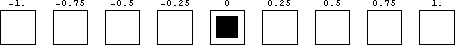There's one very straightforward way to rotate this square, which looks like:In this case we have held the z coordinate of each point fixed and rotated the points in the x and y directions. What if we want to do it differently, however, holding x fixed and rotating in the y and z directions? We can picture what that looks like in 3D; imagine taking a flat square like the one in the first of the two images above and spinning it around a horizontal (x) axis. What would that look like to a resident of Flatland? As we rotate the square up out of his plane he would go from seeing a square to just seeing a line segment. At each slice z the rotated square would look like a horizontal line segment, but the y-coordinate of the line segment would vary with z, as shown here.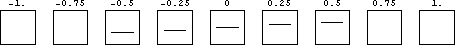What if we had started with a square lying in the yz plane? Before rotation it would have looked likeOnce again we wish to rotate this square in the yz plane. To see what this looks like we can picture this square before and after rotation in three dimensions:Since our 2D slices are at constant z, we can see that the first slice will just be a point. Subsequent slices will be vertical line segments that grow in size for a while, stay the same size while moving downwards, and finally shrink back down to a point, like so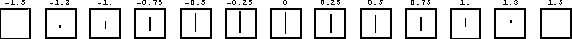(Bear in mind that the z axis points out of the page in the 3D plot, so the slices start at the back and move forwards.) It is far from intuitive to look at the plot above and recognize it as a square but hopefully by connecting it to the 3D plot you can see that it is.

Now that we've seen how to use slices to represent something we could have pictured anyway, let's try a harder problem, namely rotating a 4D hypercube.Once again there's a very straightforward way we can do it, which is to rotate each of the cubes we see. For exampleIn this case we have held the y and a coordinates of each point fixed and rotated them in x and z. What if we want to do it the other way around, though, holding x and z fixed and rotating in y and a? In other words we want to rotate into the fourth dimension. The key to figuring out what this will look like is to realize that the x and z coordinates of each point are unaffected and unimportant. At any fixed value of y and a, meaning a particular height in one of the slices shown above, we initially have a horizontal square. Each point in that square has the same initial y and a coordinates and will thus have the same post-rotation y and a coordinates as each other point in the square. In other words the square will rotate together, so this case is exactly like the 2D square we rotated above, only with each point expanded out to a horizontal square.I could go on to consider even more complicated cases, like taking the rotated square above and rotating it in the x and a directions, but I think this is enough to convey the basic idea. When I was younger and had more free time I would sometimes spend hours picturing what different 4D and 5D objects looked like rotated in different ways, and I would heartily encourage others to do the same.

### 5D and Beyond

The same basic method we have been using so far to represent 4D objects can be extended to represent objects in five or more dimensions. To represent a 5D object you can once again draw 3D slices, only now instead of a row of slices you have a grid of slices representing two additional dimensions. For example, consider a 5D hypercube. By that I simply mean the set of all points for which all the coordinates lie in the range from -1 to 1:Each point in this 5D space is represented by five numbers: its x, y, and z coordinates in the 3D plots and two other coordinates, call them a and b, representing the fourth and fifth dimensions. The numbers above the plots represent the values of a and b for each plot.

How many dimensions can you represent this way? It depends on how hard you want to work at it. Four is easy because you only need to draw in 2D—a 2D grid of 2D images. Five isn't bad if you can draw 3D shapes, as in the cube example above. To represent a 6D image you could use a 3D grid of 3D images. I can picture that in my head, but it's nontrivial to draw and if you did most of your images would probably be hidden from view anyway. Is it possible to represent more than six dimensions with this method? Actually it is, but it gets a bit messy. We've just seen how to use a set of N-dimensional drawings to represent an image in more than N dimensions, up to N+3. We could apply that trick repeatedly to generate higher dimensions. For example we could use a 2D grid of 3D images to represent a 5D object, and then draw a 2D grid of such groupings to represent a 7D object. In this way you can in principle show an arbitrarily high number of dimensions. In practice I've found that my ability to picture anything more than very simple shapes drops off quite quickly after 5D or 6D.

### Conclusions

There are endless other examples I could give of different shapes, rotations, combinations of shapes, questions to answer, and so on, but hopefully this has been enough to give you the basic idea and enough information to start playing with these images yourself if you're interested. As with any visualization technique I suspect there's nothing that you could see this way that you couldn't in principle prove algebraically without using pictures. However, as anyone who has solved a lot of math problems knows, a lot of things can be seen in an instant with a good picture that would be hard to derive and even harder to understand without one.

Is this useful for anything? It can be. In my particular field of high energy theoretical physics we often deal with systems in four dimensions (3 space and 1 time) or more, e.g. in theories like string theory where there are supposedly 9 or 10 spatial dimensions. I have often found that certain problems were easier to work on if I started out with a mental image that used this technique of slices. However, my primary interest in doing this is not practical applications, but fun. Enjoy.Gary and Kenny Felder's Math and Physics Help Home Page
`www.felderbooks.com/papers`Send comments or questions to the author